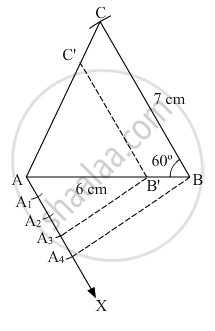# Construct a triangle ABC with BC = 7 cm, ∠B = 60° and AB = 6 cm. Construct another triangle whose sides are 3/4 times the corresponding sides of ∆ABC. - Mathematics

Construct a triangle ABC with BC = 7 cm, ∠B = 60° and AB = 6 cm. Construct another triangle whose sides are 3/4 times the corresponding sides of ∆ABC.

#### Solution

Given: A ABC with ​ BC=7 cm, B=60° and AB=6 cm

Steps of Construction

1. Draw a line segment AB = 6 cm.

2. With B as centre, draw B=60°.

3. With B as centre and radius BC = 7 cm, draw an arc.

4. Join AC to obtain ABC.

5. Below AB, make an acute angle BAX.

6. Along AX, mark off four points  A1, A2, A3 and A4 such that AA1=A1A2=A2A3=A3A4

7. Join A4B

8. From A3, draw A3B'A4B.

9. From B', draw B'C'BC.

Thus, AB'C' is the required triangle with each of its side 3/4 times the size of the corresponding sides of ABC.Concept: Division of a Line Segment
Is there an error in this question or solution?
2014-2015 (March) Delhi Set 1

Share